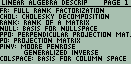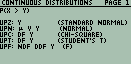## Scott Hyde

ID:1551
First Name:Scott
Last Name:Hyde
 Linalg (Linear Algebra) 1.0   (details) 49/50 48 ENG   240KB/6KBImplements linear algebra functions not built into the 48G/GX or 49/50. Includes factorization commands (Cholesky factorization, Full-Rank factorization), vector space tools (Null Space, Column Space, Eigen-space), symbolic matrix building commands, Projection Operator commands, Matrix Generation commands (including the Hilbert matrix and its inverse, the duplication matrix, the commutation matrix, and others), miscellaneous linear algebra commands (rank, pseudo-inverse (or generalized inverse), Kronecker product, matrix exponentiation, and others). By Scott Hyde. 2017/02/27
 Upper Tail Probabilities 1.0   (details) 48 ENG   203KB/4KBImplements the calculation of upper tail probabilities for a number of distributions, including the normal, chi-squared, Snedecor's F, Student's t, the Binomial, the Negative Binomial, and the Poisson distribution. Extends the usable degrees of freedom for the F and t distribution by using large-sample normal approximations. Calculation of upper-tail probabilities of the Binomial, Negative Binomial, and the Poisson distribution use relationships between them and the F or t distribution. By Scott Hyde. 2005/05/01